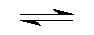## Hexa Aqua Magnesium(II+) Cation Complex Hydrolise by Aris Kaksis  Department of medical Biochemistry, Riga Stradin University RSU

At equilibrium, the following equilibrium equation is established with its conjugate base:
Mg2+(H2O)6 (aq)      +      H2O (l)Mg2+(H2O)5(OH-) (aq)   + H3O+ (aq)
acid                                                                           conjugate base
The acid and its conjugate base have different colours. At low pH values the concentration of H3O+ is high and so
the equilibrium position lies to the left. The equilibrium solution has the colour A. At high pH values, the
concentration of H3O+ is low - the equilibrium position thus lies to the right and the equilibrium solution has colour B.

blue (Acid)  pHhydrolize=11.42            red (Base)
MgOH26acdSM.skc     MgOH26acdSM.tgf     MgOH2acdSM.mol

We can apply equilibrium law to Brønsted equilibria - in general for a weak acid magnesium complex:
Keq = ( [H3O+]·[ Mg2+(H2O)5(OH-)] / ([Mg2+(H2O)6]•[ H2O]) ) eq
Keq is known as the Brønsted equilibria constant. The pH for hydrolyse is calculated at this point when:
[ Mg2+(H2O)5(OH-)]   =   [Mg2+(H2O)6]
So from equation: Keq = [H3O+] / [ H2O] =10 -11.42/ [ H2O] = 10 -11.42/ 55.33 = 10 -13.19
Keq = [H3O+] /  [ H2O] ; Keq•[ H2O] = [H3O+] =10 -11.42 ;  -log(Keq•[ H2O]) = 11.42 = pH = -log([H3O+])
The pH of the solution at its equilibrium point is called the pH and is the pH at which half of the magnesium is in its
acid form and the other half in the form of its conjugate base.
At a low pH<11.42, a weak acid aqua complex of magnesium ion is almost entirely in the [Mg2+(H2O)6] form, the
concentration of which predominates. As the pH increases pH>11.42 - the concentration of [Mg2+(H2O)6] decreases
and the equilibrium is pushed to the right to conjugate base [ Mg2+(H2O)5(OH-)] form, the concentration of which predominates.

Aqua complex of magnesium

We can apply equilibrium law to complexe stability constant equilibria - in general for a acid form magnesium cation:
Mg2+(aq)      +   OH- (aq)Mg2+(OH-)(aq)
blue (Acid)                                                             red (Base)
Kstab=[ Mg2+(OH-)]/([Mg2+]•[ OH-]) =102.58= ([ Mg2+(OH-)][H3O+]) / ([Mg2+]•[10-14])
Kstab is known as the Complexe stability constant. The pH for hydrolyse is calculated at this point when:
[ Mg2+(OH-)]   =   [Mg2+]
So from equation: Kstab = [H3O+] / 10-14 =10 2.58
Kstab•10-14 = [H3O+] =10 -11.42 ;  -log(Keq•10-14) = 11.42 = pH = -log([H3O+])
The pH of the solution at its equilibrium point is called the pH and is the pH at which half of the magnesium is in its
conjugate base and the other half in the form of its acid form.

Complex maker of magnesium cation
At a low pH<11.42, a weak acid aqua complex of magnesium ion is almost entirely in the Mg2+ form free cation, the
concentration of which predominates. As the pH increases pH>11.42 - the concentration of [Mg2+] decreases and the
equilibrium is pushed to the right to conjugate base    [Mg2+(OH-)] form, the concentration of which predominates.

Literature

Handbook of  Chemical Equilibria in Analytical Chemistry. S.Kortly, L.Sucha. New York , Ellis Horwood LTD. 1985. 414.p.